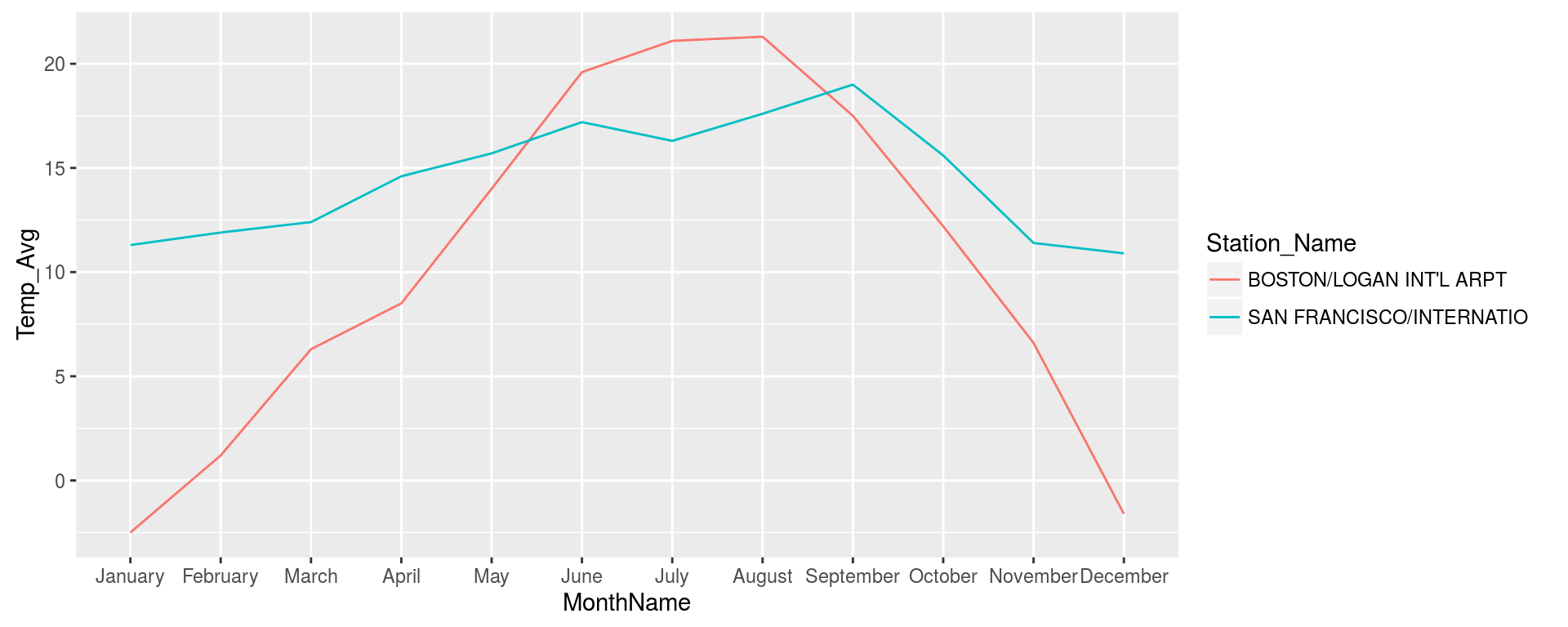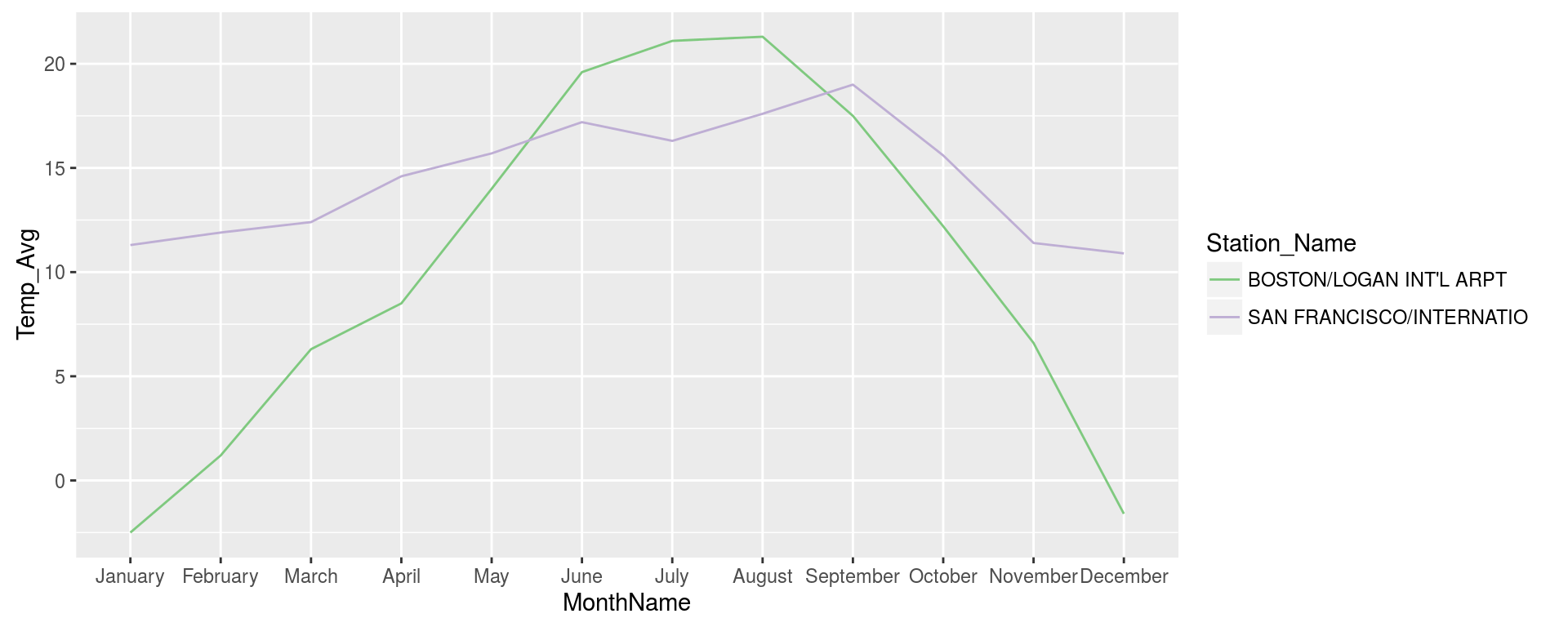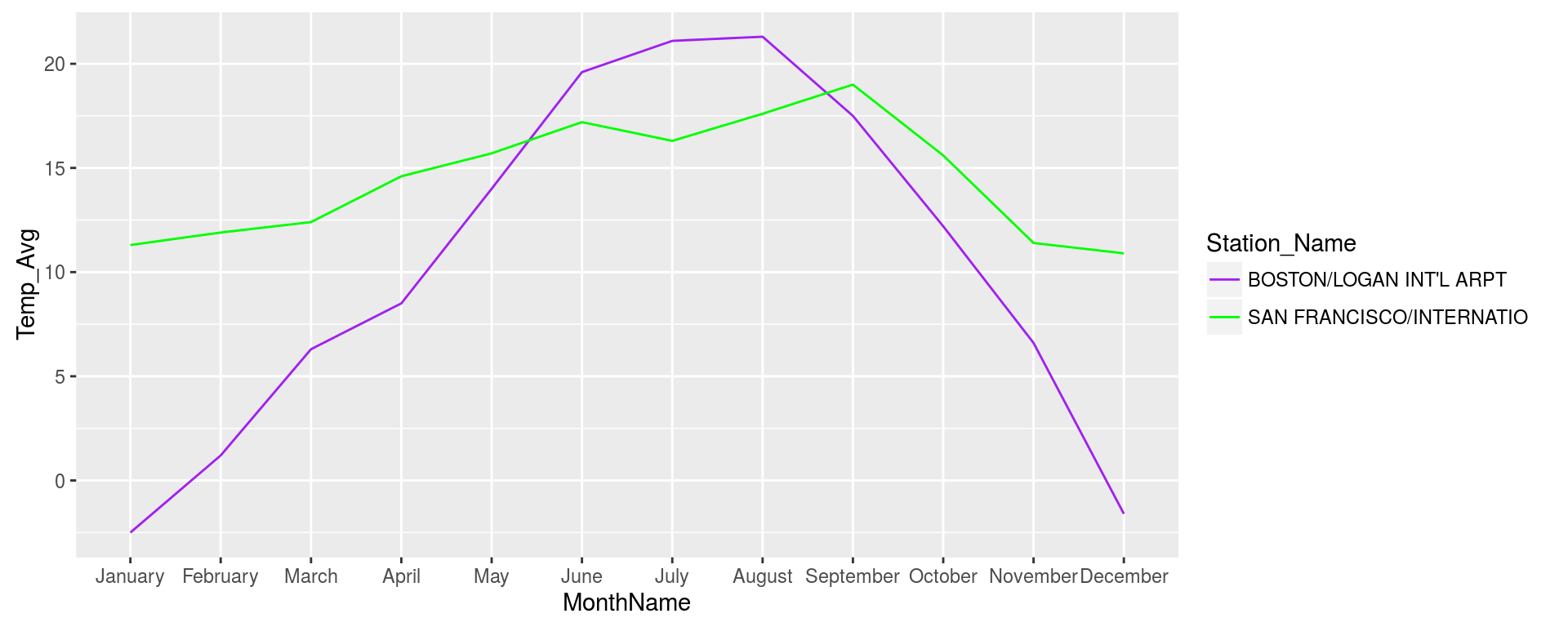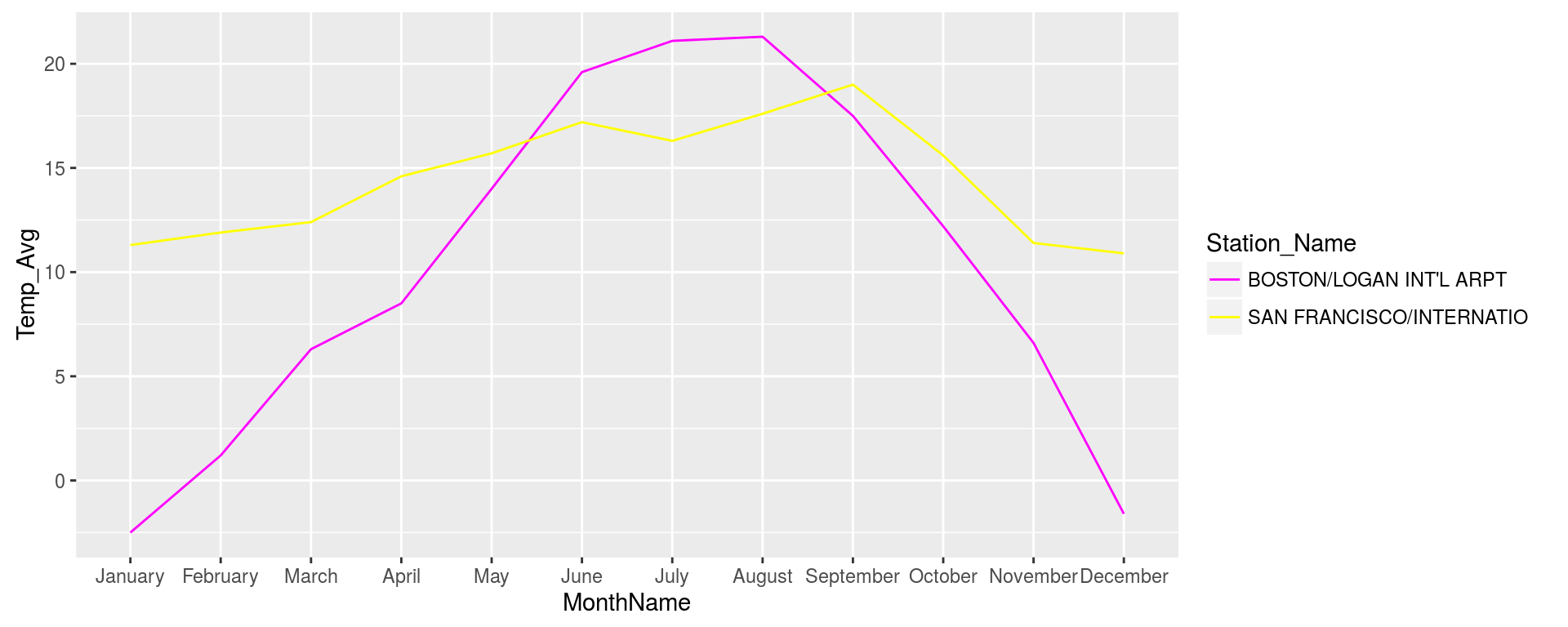# Setup

From Session 3, reading in and manipulating data from the temperature data set.

``````require(readr)
require(tidyr)
require(dplyr)
require(ggplot2)
require(easyGgplot2)

tmax = read_tsv("tmax_worldTemp/data.txt", col_names = F, comment = "%")
tmin = read_tsv("tmin_worldTemp/data.txt", col_names = F, comment = "%")
tavg = read_tsv("tavg_worldTemp/data.txt", col_names = F, comment = "%")

tmax_meta = read_tsv("tmax_worldTemp/site_detail.txt", col_names = F, comment = "%")
tmin_meta = read_tsv("tmin_worldTemp/site_detail.txt", col_names = F, comment = "%")
tavg_meta = read_tsv("tavg_worldTemp/site_detail.txt", col_names = F, comment = "%")

colnames(tmax) = c("Station ID", "Series Number", "Date", "Temperature (C)", "Uncertainty (C)","Observations","Time of Observation")
colnames(tmin) = c("Station ID", "Series Number", "Date", "Temperature (C)", "Uncertainty (C)","Observations","Time of Observation")
colnames(tavg) = c("Station ID", "Series Number", "Date", "Temperature (C)", "Uncertainty (C)","Observations","Time of Observation")

colnames(tmax) = gsub(" ", "_", colnames(tmax))
colnames(tmin) = gsub(" ", "_", colnames(tmin))
colnames(tavg) = gsub(" ", "_", colnames(tavg))

metaCols = "Station ID, Station Name, Latitude, Longitude, Elevation (m), Lat. Uncertainty, Long. Uncertainty, Elev. Uncertainty (m), Country, State / Province Code, County, Time Zone, WMO ID, Coop ID, WBAN ID, ICAO ID, # of Relocations, # Suggested Relocations, # of Sources, Hash"

colnames(tmax_meta) = gsub(" ", "_",unlist(strsplit(metaCols, ", ")))
colnames(tmin_meta) = gsub(" ", "_",unlist(strsplit(metaCols, ", ")))
colnames(tavg_meta) = gsub(" ", "_",unlist(strsplit(metaCols, ", ")))

tmax_meta = select(tmax_meta, one_of("Station_ID", "Station_Name", "Latitude", "Longitude", "Country", "State_/_Province_Code"))

tmax_sel = select(tmax, one_of("Station_ID", "Date", "Temperature_(C)"))
tmin_sel = select(tmin, one_of("Station_ID", "Date", "Temperature_(C)"))
tavg_sel = select(tavg, one_of("Station_ID", "Date", "Temperature_(C)"))

colnames(tmax_sel) = "Temp_Max"
colnames(tmin_sel) = "Temp_Min"
colnames(tavg_sel) = "Temp_Avg"

temps = left_join(tmax_sel, tmin_sel, by = c("Station_ID", "Date"))
temps = left_join(temps, tavg_sel, by = c("Station_ID", "Date"))

temps\$Date = as.character(temps\$Date)
temps = separate(temps, Date, c("Year", "Month"), sep = c("\\."))

temps\$Year = as.numeric(temps\$Year)
temps\$Month = as.numeric(temps\$Month)

temps = mutate(temps, Month = round((Month/1000) * 12 + 0.5 ))
temps = mutate(temps, MonthName = month.name[Month])

temps = left_join(temps, tmax_meta, by = "Station_ID")

temps_usa = filter(temps, Country == "United States")
temps_usa\$MonthName = factor(temps_usa\$MonthName, levels = month.name)

temps_usa_sanBos = filter(temps_usa, Station_Name %in% c("SAN FRANCISCO/INTERNATIO", "BOSTON/LOGAN INT'L ARPT"))``````

# Tweaking Graphs

When it comes to plotting in R you will be doing a lot of tweaking of graphing parameters just like you would in a graphical editor but the tweaking is done by writing more code which will probably take some taking use to. Below I have some common examples of tweaking graphs using examples from Session 3. ##Line Plot Below will graph the San Francisco and Boston dataset and we will give it a filtered dataset so that we are only graphing the year 2000. Again in ggplot call the first argument is the data we will be graphing, next comes some descriptions for the graph that comes in the ggplot2 supplied function aes (again stands for aesthetics), here we give it column names of what we want to graph, so we want the months to be the x axis and y axis to be the average temperature, and we want to group and color by Station_Name

``````plotObj = ggplot(filter(temps_usa_sanBos, Year == 2000), aes(x = MonthName, y = Temp_Avg, group = Station_Name, color = Station_Name) )
plotObj = plotObj + geom_line()
#now lets call print(plotObj) to generate the plot
print(plotObj)``````## Chaning the colors

By default ggplot2 will choose some colors for you, but these aren’t always the best colors to use. They have a wide variety of functions that can be used to change the colors being used. One among is the the scale_color_brewer function which is used to modify anything being colored by the color setting in aes() and the brewer stands for ColorBrewer colors, which is a great site for choosing color paletes.

``````plotObj = ggplot(filter(temps_usa_sanBos, Year == 2000), aes(x = MonthName, y = Temp_Avg, group = Station_Name, color = Station_Name) )
plotObj = plotObj + geom_line()
plotObj = plotObj + scale_color_brewer(type = "qual")
#now lets call print(plotObj) to generate the plot
print(plotObj)``````## Supplying own colors

You can use `scale_color_manual` function to give your own colors by setting `values` in `scale_color_manual` to a vector of colors. R has a bunch of named colors and you can give one of these names to change the colors.

``````plotObj = ggplot(filter(temps_usa_sanBos, Year == 2000), aes(x = MonthName, y = Temp_Avg, group = Station_Name, color = Station_Name) )
plotObj = plotObj + geom_line()
plotObj = plotObj + scale_color_manual(values = c("purple", "green"))
#now lets call print(plotObj) to generate the plot
print(plotObj)``````You can also give the hex number of a color if you know it.

``````plotObj = ggplot(filter(temps_usa_sanBos, Year == 2000), aes(x = MonthName, y = Temp_Avg, group = Station_Name, color = Station_Name) )
plotObj = plotObj + geom_line()
plotObj = plotObj + scale_color_manual(values = c("#FF00FF", "#FFFF00"))
#now lets call print(plotObj) to generate the plot
print(plotObj)``````There is also a packaged called RColorBrewer that implements ColorBrewer color paletes for you, you can see all available paletes with `RColorBrewer::display.brewer.all()` and then choose a palete and the number of colors needed by using `RColorBrewer::brewer.pal()`, my favorite is `"Dark2"`.

``````plotObj = ggplot(filter(temps_usa_sanBos, Year == 2000), aes(x = MonthName, y = Temp_Avg, group = Station_Name, color = Station_Name) )
plotObj = plotObj + geom_line()
plotObj = plotObj + scale_color_manual(values = RColorBrewer::brewer.pal(3, "Dark2"))
#now lets call print(plotObj) to generate the plot
print(plotObj)``````